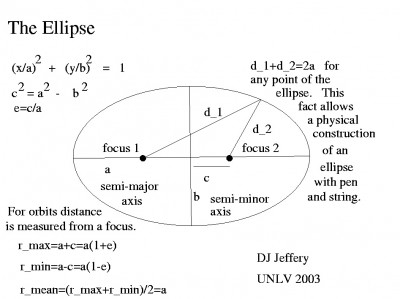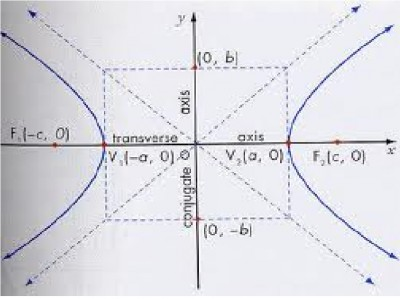# Difference between Hyperbola and Ellipse

In mathematics we encounter two very well known conic sections which are hyperbola and ellipse. These curves are formed as a result of intersection between the right circular cone surface and a plane surface. It is important to differentiate the two in order to clearly understand their underlying concepts.

The major difference between hyperbola and ellipse can be seen in their shapes. A hyperbola consists of two curves that never meet  each other at any point while an ellipse is a closed figure such that its start and end points meet each other. This also implies that since the ellipse is a closed figure so we can also find its length along the line forming the closed figure but we cannot find the length or perimeter of an open figure like hyperbola.

In addition to this, these two also differ in their measure of eccentricities. As the measure of eccentricity is the deviation from being circular so the eccentricity of an ellipse falls between 0 and 1 while the eccentricity of a hyperbola is greater then 1.

Another significant difference is the position of the directrix. When we talk about the ellipse, the position of the directrix is always external to the semi-major axis. While in case of hyperbola the position of the directerix is inside the semi major axis. Therefore the ratio of the distance of some point on the ellipse from focus to distance from directrix will always be less than 1 but in case of hyperbola it will be greater then 1.

Also their standard equation is also different from each other.

There is another very significant difference which is in their graphs. In case of ellipse the curve is intersected by the perpendicular bisector of the major-axis which is not the case in hyperbola.

### Instructions

• 1

Ellipse:

While intersecting a cone with a plane surface ellipse is formed when the set of all points in a plane is such that the sum of their distances from two fixed points is unvarying. These fixed points are called foci.

Therefore the standard equation of an ellipse is (x/a)^2 + (y/b)^2=1.

- Image Courtesy: physics.unlv.edu• 2

Hyperbola:

A hyperbola is formed when the set of all points in the plane are such that the difference of their distance from the two fixed points is unvarying. These fixed points are the foci.

A hyperbola is an open figure with two branches running infinitely in opposite directions and they are the mirror images of each other.

Therefore the standard equation of an ellipse is (x

/a)^2 - (y/b)^2=1.

- Image Courtesy: algebra1help.com•